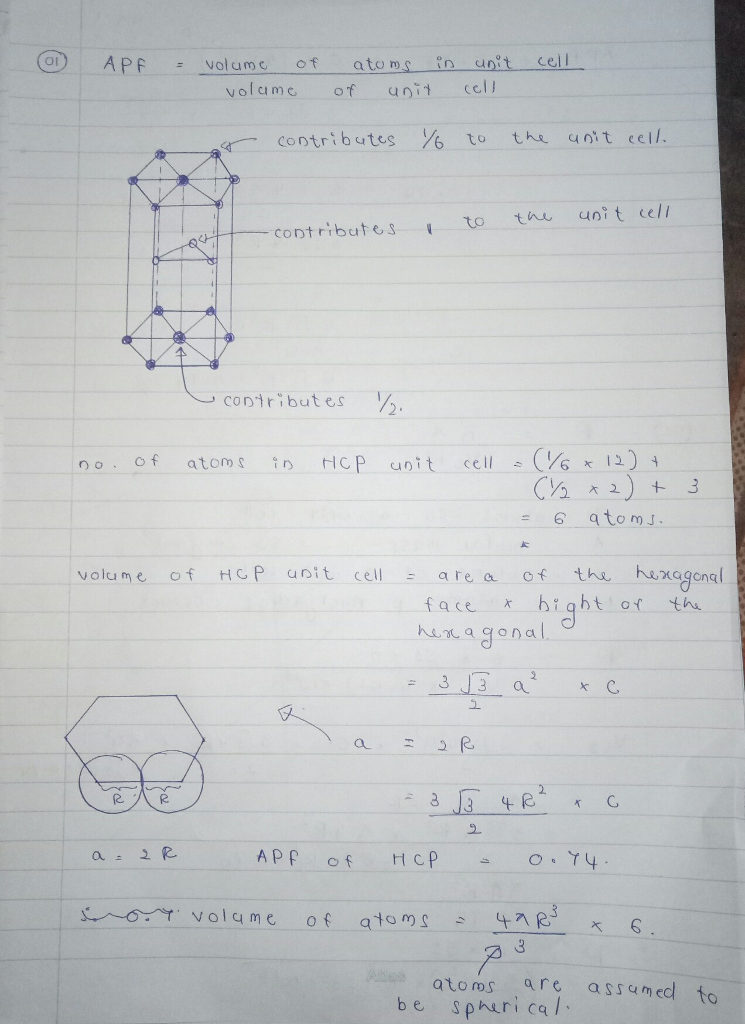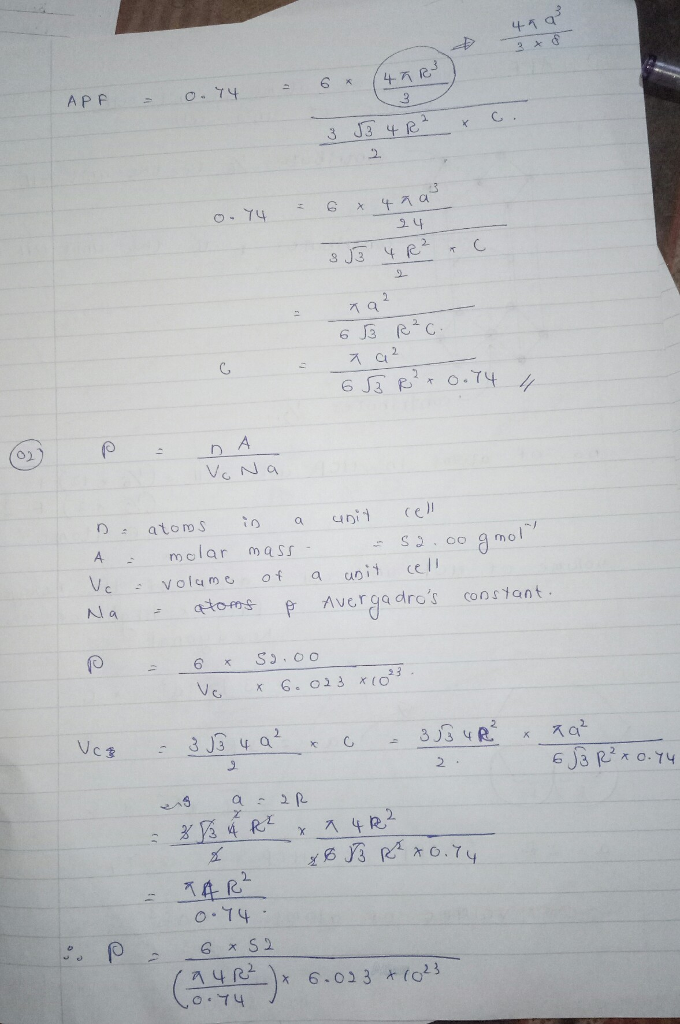# Homework Solution: Given a hexagonal close packed crystal structure (depicted below) calculate the…

Given a hexagonal close packed crystal structure (depicted below) calculate the height of the hexagonal prism, c, in terms of R and a. Recall: APF = volume of atoms in unit cell/volume of unit cell Area of Hexagon = 3 squareroot 3/2 a^2 Volume of prism area of base * c a=2R Chromium forms a hexagonal close packed crystal structure. Determine the theoretical density of chromium rho = nA/V_cN_a